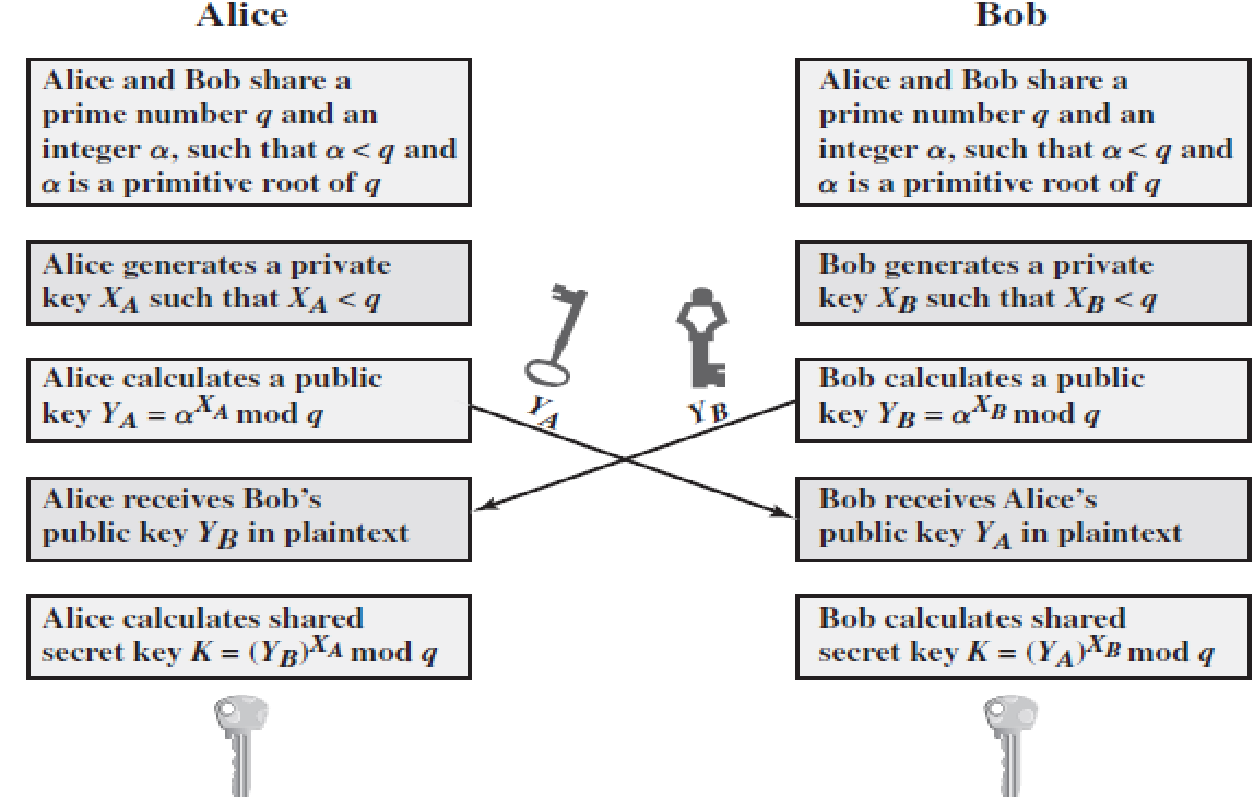# Explain Diffie Hellman key exchange algorithm ?

• Diffie–Hellman key exchange is a method of securely exchanging cryptographic keys over a public channel
• Purpose is to enable two users to securely exchange a key that can then be used for subsequent symmetric encryption of messages
• The algorithm is limited to the exchange of secret values
• Its effectiveness depends on the difficulty of computing discrete logarithms

following way. Recall from Chapter 2 that a primitive root of a prime number p is one whose powers modulo p generate all the integers from 1 to p -  1. That is, if a  is a primitive root of the prime number p , then the numbers

a  mod p , a2  mod p , . . .  , ap-1  mod p

are distinct and consist of the integers from 1 through p -  1 in some permutation. For any integer b  and a primitive root a of prime number p , we can find a unique exponent i such that

b = ai  (mod p ) where 0 ≤  i ≤ (p -  1)

The exponent i  is referred to as the discrete logarithm  of b  for the base a , mod p .

We express this value as d loga,p (b ).

Diffie-Hellman Key Exchange algorithmDiffie-Hellman Key Exchange algorithm

• For this scheme, there are two publicly known numbers: a prime number q  and an integer a  that is a primitive root of q
• User A selects a random integer XA < q and computes YA = aXA mod q.
• Similarly, user B independently selects a random integer XB < q and computes YB = aXB mod q.
• Each side keeps the X value private and makes the Y value available publicly to the other side. Thus, XA is A’s private key and YA is A’s corresponding public key, and similarly for B.
• User A computes the key as K = (YB)XA mod q    and user B computes the key as K = (YA)XB mod q

These two calculations of K can be shown to produce identical results:

K = (YB)XA mod q   (this K is the secret key calculated by Alice, since XB is known only to Alice)

= (aXB mod q)XA mod q

= (aXB)XA mod q                 by the rules of modular arithmetic

= aXBXA mod q

= (aXA)XB mod q

= (aXA mod q)XB mod q

= (YA)XB mod q  (here K is secret key calculated by Bob, since XB is known only to Bob)

Man in-the-middle Attack:

• The Diffie –Hellman key exchange protocol is insecure against a man-in-the-middle attack.
• The protocol is vulnerable to such an attack because it does not authenticate the participants.
• This vulnerability can be overcomed with the use of digital signatures and public- key certificates.
• The attack is described as follows: